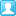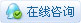##账号 自动登录 找回密码 密码 立即注册
 搜索

# [曲线] 计算和生成椭圆弧已领礼包: 40个财富等级: 招财进宝发表于 2021-1-12 12:46:36 | 显示全部楼层 |阅读模式

### 马上注册，结交更多好友，享用更多功能，让你轻松玩转社区。

×

Calculate and entmake elliptical arcs (with LISP sample)

ical arcs, group code 41 is described as the "start parameter".
However, its association with known values for the arc is difficult to
determine. How is this value derived?

An elliptical arc is a special version of an arc that follows the
eccentricity of the ellipse. One way to generate this type of arc is to find
the parametric normal of the starting point. To do so, you must specify a
start angle that is different from the actual start angle of the drawn arc.
Group code 41 contains this parametric angle expressed in

What Is the Parametric Angle?

The parametric angle is generated from two concentric circles whose center is
the center of the ellipse and whose radii are the major and minor axes,
respectively. Every point of the ellipse lies either between or on these two
circles, and each elliptical point can be defined by a unique relation to
them. To discover this relationship, draw a line perpendicular to the major
axis from a point on the ellipse to the closest intersection with the circle
described by the major axis. Then do the same with the minor axis, starting
from the elliptical point and drawing perpendicular to the minor axis until
the line intersects the circle described by the minor axis. The two points of
intersection with the circles are colinear with the center of the ellipse.
The angle between the line containing these three points and the major axis
is the parametric angle specified by group code 41.

How Do I Calculate It from the True Start Angle?

To calculate the parametric angle from the true start angle, you must
first find the start point on the ellipse. This requires a simultaneous
solution to the equations for the line and the ellipse. In this example we
assume that the major axis of the ellipse lies on the x-axis with the origin
at the center of the ellipse. When this point is found, you
can use its y-value and the minor axis to solve the equation for the circle
whose radius is the minor axis value and whose center is the center of the
ellipse. This will provide the x,y point on the circle that dictates the
parametric angle from the center of the ellipse.

Below is an AutoLisp example that demonstrates how to use trigonometric

functions to determine the parametric angle:
LISP源码如下：已领礼包: 4个财富等级: 恭喜发财发表于 2022-8-2 23:15:17 | 显示全部楼层
 看看，也许能用到发表于 2023-4-24 10:19:02 | 显示全部楼层
 谢谢楼主的分享发表于 2023-5-20 21:47:02 | 显示全部楼层
 分数太低学习不到，下次再来！

 本版积分规则 回帖并转播 回帖后跳转到最后一页|申请友链|Archiver|手机版|小黑屋|辽公网安备|晓东CAD家园 ( 辽ICP备15016793号 )

GMT+8, 2023-12-8 07:52 , Processed in 0.388570 second(s), 25 queries , Gzip On.